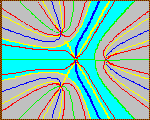# THEORY OF EQUATIONS

## 2002 Edition

#### Introduction (HTML)

Chapter 1 College Algebra

1.1 The Real Number System
1.2 A little History of Real and Complex Numbers
1.3 Complex Numbers
Lesson 2 (PDF 105KB) 1.4 Polynomial Arithmetic
1.5 Degree
1.6 The division Algorithm
1.7 Factors and Roots
Lesson 3 (PDF 146KB) 1.8 Greatest Common Divisor
1.9 Unique Factorization
1.10 Formal Differentiation of Polynomials
1.11 Test for Multiple Roots
1.12 Partial Fraction Decomposition
1.13 The Resultant
New Polynomial GCDs by Linear Algebra
Appendix to Chapter 1, (PDF 57KB)

Chapter 2 Numerical Analysis

2.1 Exact and Approximate Numbers
2.2 Numerical Algorithms
2.3 Maple Programming and Evaluation of Polynomials
2.4 Taylor's Series and Horner's Process
2.5 Synthetic Division
2.6 Graphing
2.7 Descartes' Rule and Newton's Bound
2.8 The Bisection Method
2.9 Horner's Method
2.10 Iteration Method
2.11 Newton's Method, Real Case
2.12 Newton's Method, Complex Case
2.13 The Newton-Barstow Algorithm
2.14 Other root finding algorithms
2.15 Polynomial Interpolation
Chapter 3 Topology 3.1 History of the Fundamental Theorem of Algebra
3.2 Gauss' Fourth Proof
3.3 Topological Proof
3.4 Analysis Proof
Lesson 8 (PDF 197KB) 3.5 Another Curve Proof
3.6 Connection between the FTA and Newton's method
3.7 Where Newton's method does not converge
3.8 Real Newton's method revisited
Chapter 4: Ancient and Modern Algebra 4.1 Solutions of Quadratic Equations
4.2 Omar Khayyam and Viete's Solutions of the cubic
4.3 History of the Cubic and Biquadratic
4.4 Algebraic solution of the cubic
4.5 Algebraic solution of the biquadratic
Lesson 10 (PDF 151KB) 4.6 Newton's Identities
4.7 More on Newton's Indentities
4.8 Symmetric Polynomials
4.9 Lagrange's Solution of the Biquadratic
4.10 Insolvability of the Quintic
Chapter 5 Number Theory 5.1 Rational Polynomials and Algebraic Numbers
5.2 Integer Polynomials
5.3 Rational Roots and Factors
5.4 Eisenstein's Irreducibility Criterion
5.5 Hand Factoring methods
5.6 Computer Factoring
5.7 Comparison of factoring algorithms
Appendix to Chapter 5 on Polynomials over the integers mod p. 1. Modular Arithmetic
2. Polynomials over Zp
3. Factoring in Zp[x]
4. Roots of Polynomials in Zn

Chapter 6 Analysis -- Elliptic Functions

6.1 Review of trigonometric and hyperbolic functions
6.2 Historical Background
6.3 The Jacobi Elliptic Functions
6.4 The inverse Jacobi Elliptic Functions
6.5 Elliptic Integrals
6.6 The Complex Theory
For an updated look at relevant Mathematica software for Elliptic Functions and integrals please see wolfram.com